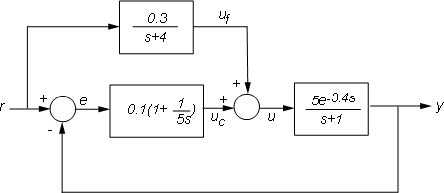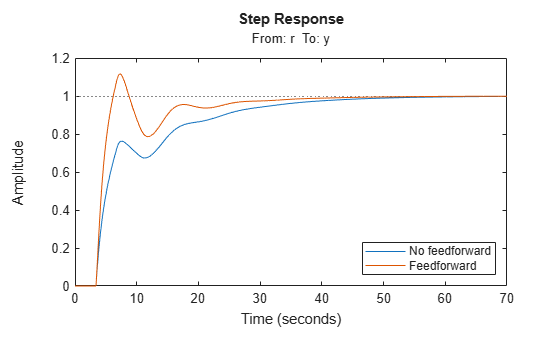## Analysis of Systems with Time Delays

You can use analysis commands such as `step`, `bode`, or `margin` to analyze systems with time delays. The software makes no approximations when performing such analysis.

For example, consider the following control loop, where the plant is modeled as first-order plus dead time:You can model the closed-loop system from `r` to `y` with the following commands:

```s = tf('s'); P = 5*exp(-3.4*s)/(s+1); C = 0.1 * (1 + 1/(5*s)); T = feedback(P*C,1);```

`T` is a state-space model with an internal delay. For more information about models with internal delays, see Closing Feedback Loops with Time Delays.

Plot the step response of `T`:

`stepplot(T)`For more complicated interconnections, you can name the input and output signals of each block and use `connect` to automatically take care of the wiring. Suppose, for example, that you want to add feedforward to the control loop of the previous model.You can derive the corresponding closed-loop model `Tff` by

```F = 0.3/(s+4); P.InputName = 'u'; P.OutputName = 'y'; C.InputName = 'e'; C.OutputName = 'uc'; F.InputName = 'r'; F.OutputName = 'uf'; Sum1 = sumblk('e','r','y','+-'); % e = r-y Sum2 = sumblk('u','uf','uc','++'); % u = uf+uc Tff = connect(P,C,F,Sum1,Sum2,'r','y');```

and compare its response with the feedback only design.

```stepplot(T,Tff) legend('No feedforward','Feedforward')```The state-space representation keeps track of the internal delays in both models.

### Considerations to Keep in Mind when Analyzing Systems with Internal Time Delays

The time and frequency responses of delay systems can look odd and suspicious to those only familiar with delay-free LTI analysis. Time responses can behave chaotically, Bode plots can exhibit gain oscillations, etc. These are not software or numerical quirks but real features of such systems. Below are a few illustrations of these phenomena.

Gain ripple:

```s = tf('s'); G = exp(-5*s)/(s+1); T = feedback(G,.5); bodemag(T)```Gain oscillations:

```G = 1 + 0.5 * exp(-3*s); bodemag(G)```Jagged step response:

```G = exp(-s) * (0.8*s^2+s+2)/(s^2+s); T = feedback(G,1); stepplot(T)```Note the rearrivals (echoes) of the initial step function.

Chaotic response:

```G = 1/(s+1) + exp(-4*s); T = feedback(1,G); stepplot(T,150)```You can use Control System Toolbox™ tools to model and analyze these and other strange-appearing artifacts of internal delays.